« A tip for laying out captioned figures in Microsoft Word Global Warming - Not Funny Anymore »

## 02/19/08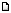03:10:53 pm, by millercommamatt, 845 words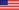Categories: General, Meteorology

### Correlation, Confidence Interval, and Sample Size

I haven't made a post to this journal in a very long time and I'm about to end that drought with something very nerdy. [WARNING - MATH AHEAD]

Lately in my research, I've been looking at correlations between data. For those of you who aren't familiar with the concept, correlation ranges between -1 and 1 and is an expression of the linear relationship between two sets of data. In English, it is an expression of how well one variable relates to another variable.

To give an example, temperature and the number of people at the beach are well correlated. When the temperatures are warm, there are typically a lot of people at the beach. When the temperatures are cold, there are typically not a lot of people at the beach. Thus, you can use temperature to predict the number of people at the beach and you can use the number of people at the beach to predict the temperature. When two things are not well correlated is basically means that knowing something about one thing doesn't give you any predictive information about the second thing. It's important to note that correlation doesn't provide any information about causation. Just because two things are correlated, does mean they affect each other in any way. To play off our earlier example, the number of people at the beach has no effect on the air temperature. For a more well written written explanation of correlation with some visual illustrations, I suggest reading the following linked Wikipedia article: Wikipeida - Correlation

Anyway, correlation statistics are based on samples of data taken from a larger set. Since the entire dataset isn't being sampled (at least for any real-world dataset) there is some uncertainty about the calculated correlation value. The confidence interval for a given correlation value is calculated as follows:

1) The correlation (r) is transformed using a Fisher's Z transformation. This is the same as: hyperbolic arctangent - arctanh(r) = Z'

2) Calculate the upper and lower bounds using the following formula: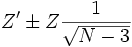,
where Z' is a product of the transformation described in step 1, N is the number of samples, and Z is a value taken from a statistical Z table depending on the confidence interval you want to use. For a 95% confidence interval (i.e. the correlation of the true dataset has a 95% chance of being within the calculated upper and lower bounds) we use a value of 1.96.

3) You take the two values calculated from step 2 and turn them back into correlation values using an inverse Fisher's Z transform. This is the same as taking the hyperbolic tangent of the values from step 2.

For the statistical reasoning behind this process, I suggest reading the following link: Confidence Interval on Pearson's Correlation

The resulting values are the upper and lower bounds for the correlation values. The true correlation for the dataset you sampled should be somewhere within those bounds. If you examine the formula you can see that the more samples you have, the more narrow your bounds are and the more certain you can be of the true correlation.

In my research, I've been working a lot recently with correlations and their confidence intervals. I started wondering exactly how the confidence interval changed for different sample sizes for a given correlation. I also wondered about the symmetry of the confidence intervals. Often in published research, you see a correlation with its confidence interval expressed as follows: 0.6±0.1. This states that the correlation is 0.6 with an upper bound of 0.7 and a lower bound of 0.5. This states that the upper and lower bounds are symmetric (different by the same amount) about the correlation value. The problem is that when you actually look how confidence intervals are calculated, you'll see that they are never truly symmetric. To illustrate this, I created the following graph for a correlation of 0.6 with 95% confidence intervals: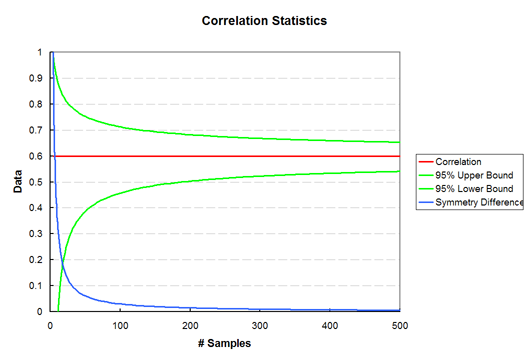If you take a look at the graph, you can see how the upper and lower correlation bounds narrow with increasing sample size. As the number of samples increases, you become more certain about what the true correlation, but never 100%. The confidence only become really narrow (0.01 or less above or below the correlation value) for obscenely large sample sizes (~100,000+).

The curve in blue is an expression of the symmetry of the correlation bounds. It's basically a plot of the difference between the upper bound and the correlation and the lower bound and the correlation. If the value is zero, the bounds are perfectly symmetric. As you can see from the graph, the symmetry is large for small sample sizes and decreases asymptotically towards zero. It's worth noting that the correlation bounds are never truly symmetric.

The question I'm currently grappling with is how symmetric is symmetric enough to where I can just write the correlation values as being such and when can I not do this?

For the education of the curious, I have versions of the above graphs for two other correlation values.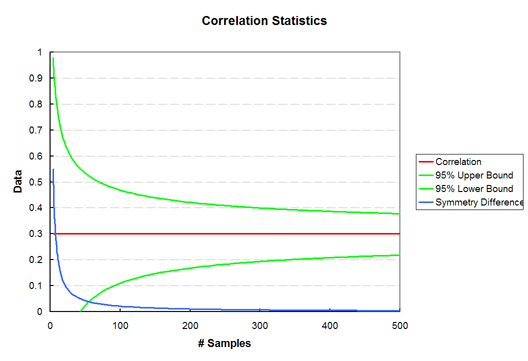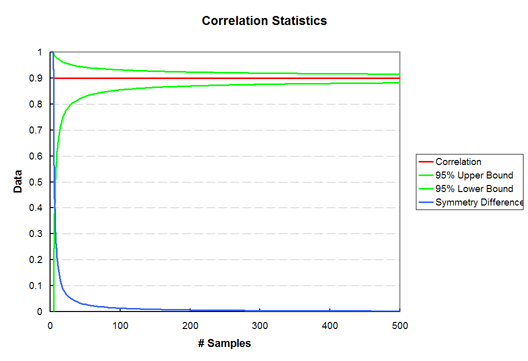It's also worth noting that the correlation bounds are wider for correlation values closer to zero for any given number of samples.

What is this thing?

This is where I rant about whatever I want. Here is where I share my thoughts, dreams, strange ideas, and general commentary on my life and the world around me.

### Search

April 2021
Sun Mon Tue Wed Thu Fri Sat
<< <   > >>
1 2 3
4 5 6 7 8 9 10
11 12 13 14 15 16 17
18 19 20 21 22 23 24
25 26 27 28 29 30